# RD Sharma Solutions for Class 8 Maths Chapter 21 Mensuration - II (Volumes and Surface Areas of a Cuboid and a Cube) Exercise 21.1

In our everyday life, we come across objects like a wooden box, a tea packet, a chalk box, a dice, a book etc. All these objects have similar shapes of a cuboid. In Exercise 21.1 of Chapter 21, we shall discuss the volume of the space region formed by a body, formula for finding the volume of a cuboid and volume of a cube. RD Sharma Class 8 Solutions have the answers to these topics which are prepared by our experts at BYJU’S keeping in mind the latest syllabus, to help students prepare for their exams. Students can refer and download the PDF from the links given below.

## Download the Pdf of RD Sharma Solutions for Class 8 Maths Exercise 21.1 Chapter 21 Mensuration – II (Volumes and Surface Areas of a Cuboid and a Cube)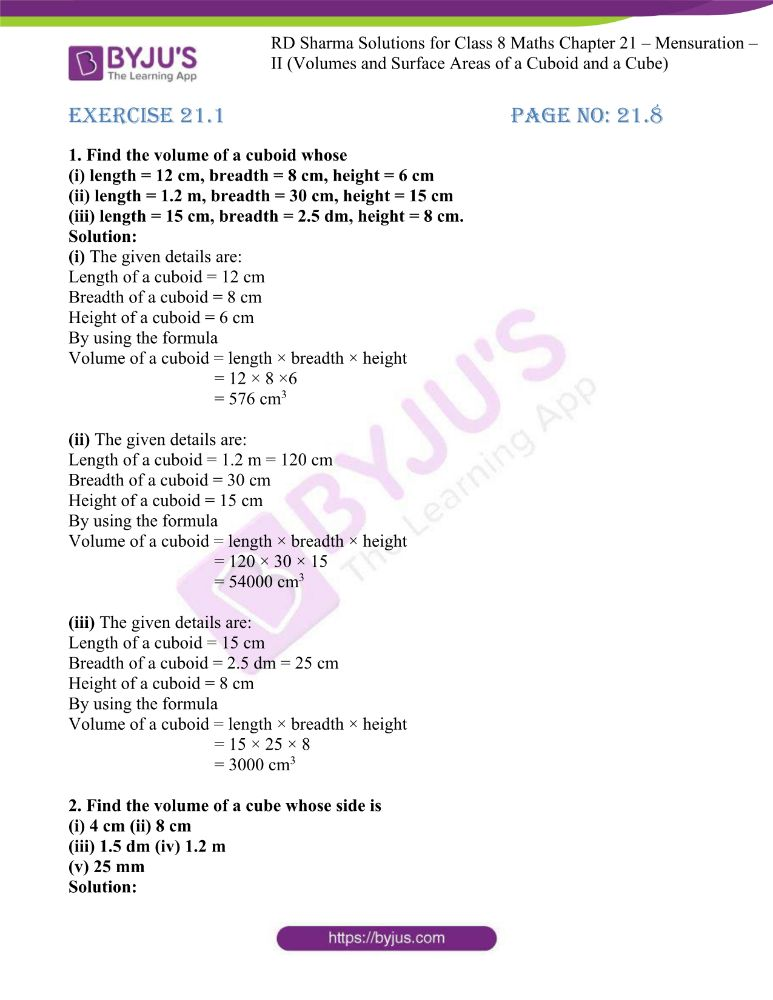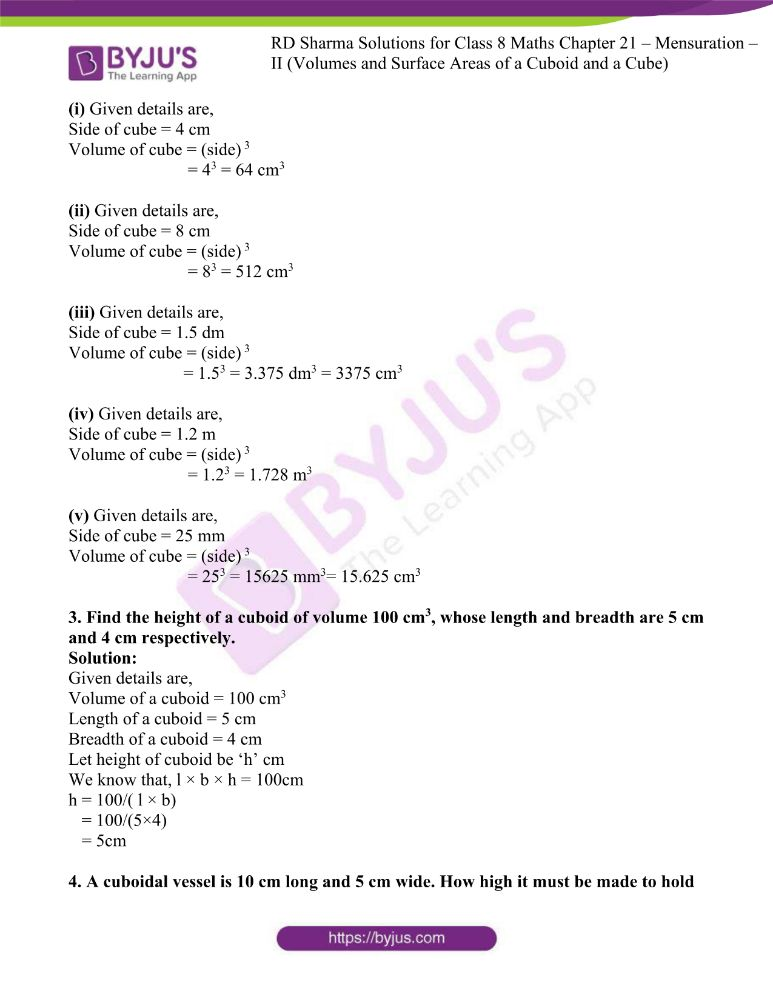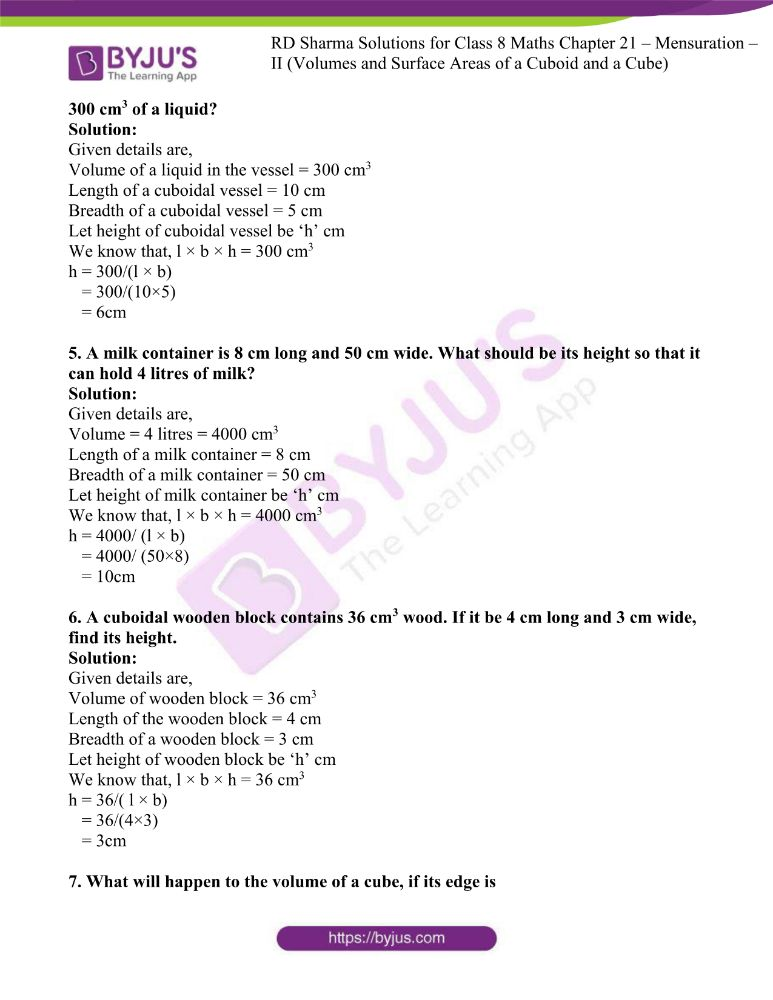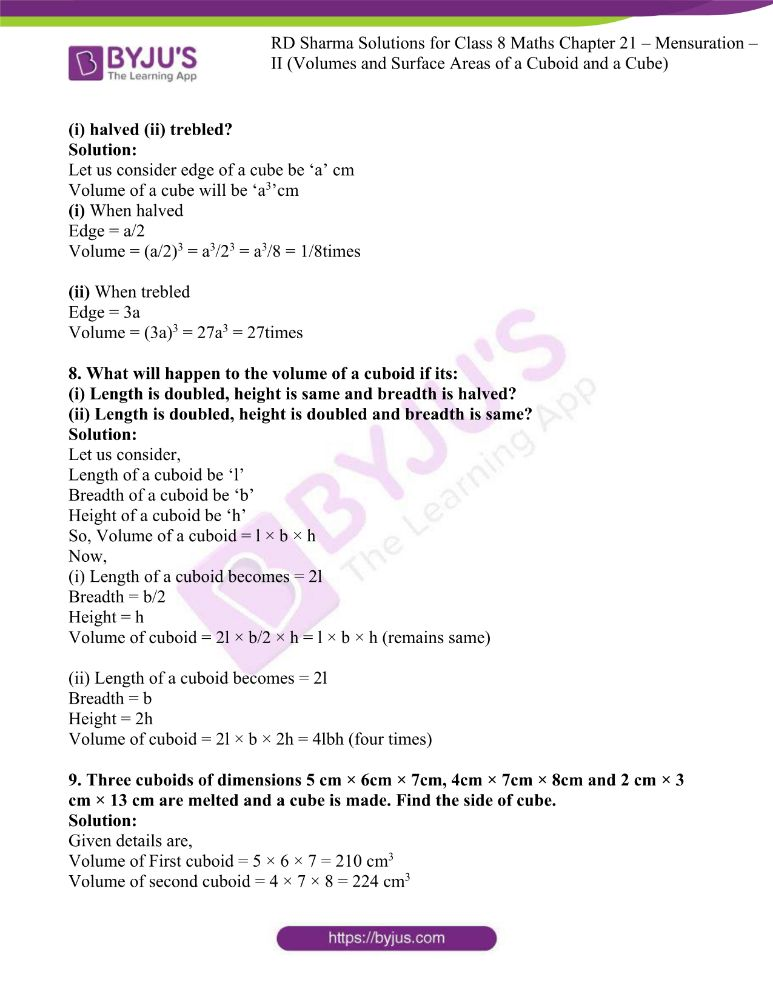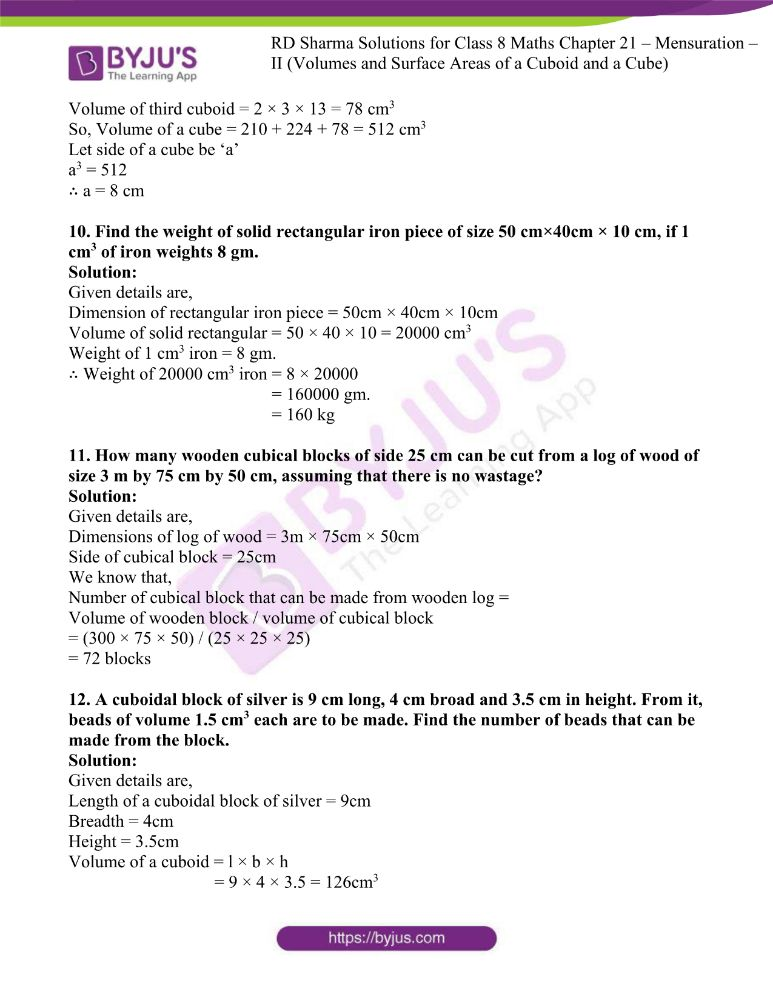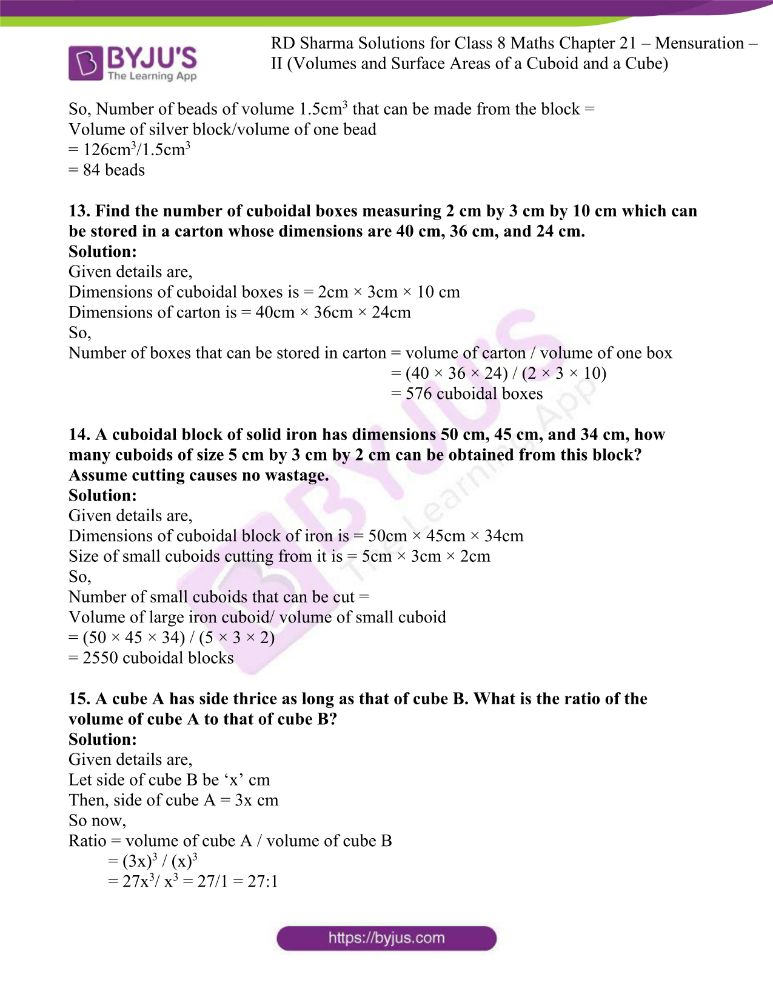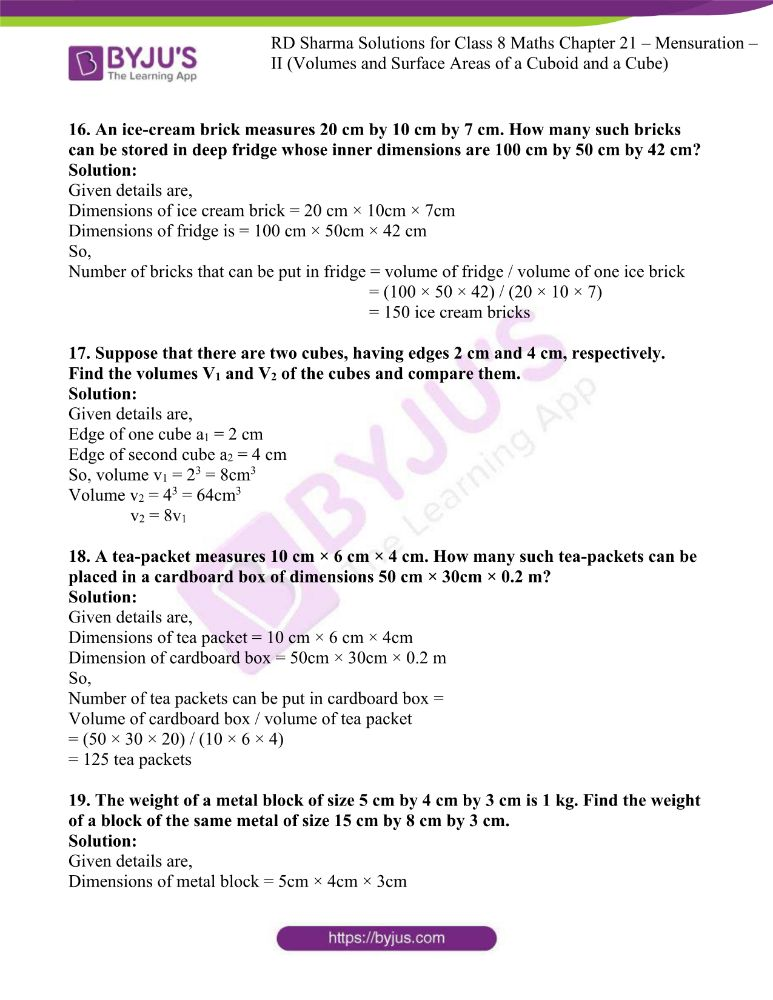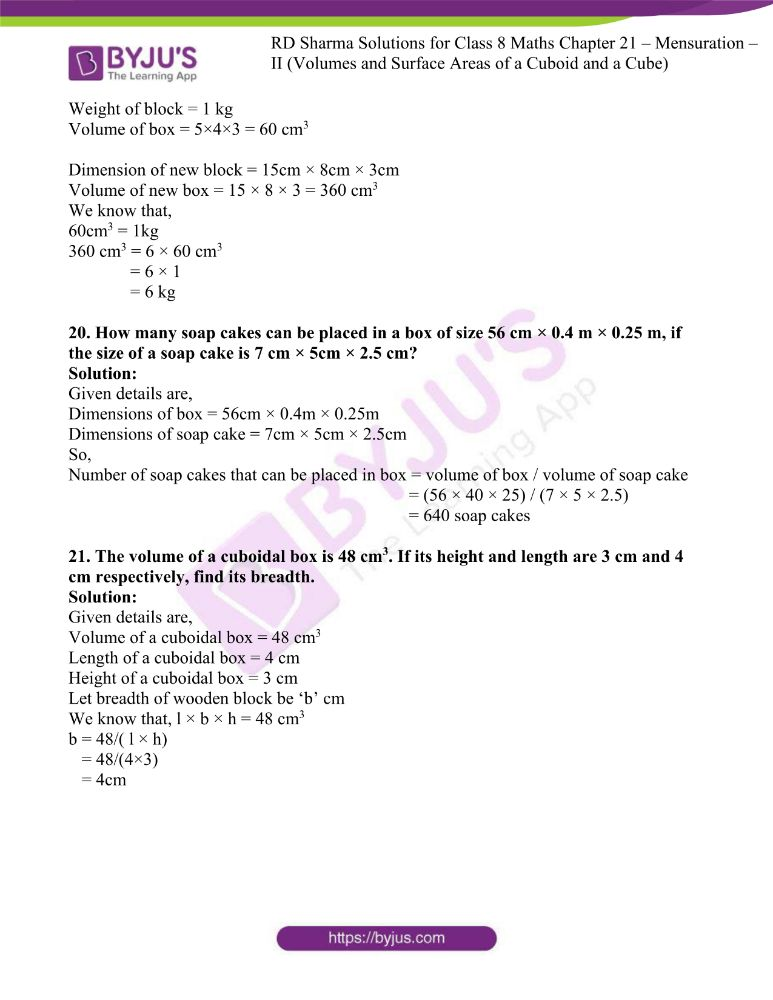### Access answers to RD Sharma Maths Solutions For Class 8 Exercise 21.1 Chapter 21 Mensuration – II (Volumes and Surface Areas of a Cuboid and a Cube)

1. Find the volume of a cuboid whose
(i) length = 12 cm, breadth = 8 cm, height = 6 cm
(ii) length = 1.2 m, breadth = 30 cm, height = 15 cm
(iii) length = 15 cm, breadth = 2.5 dm, height = 8 cm.

Solution:

(i) The given details are:

Length of a cuboid = 12 cm

Breadth of a cuboid = 8 cm

Height of a cuboid = 6 cm

By using the formula

Volume of a cuboid = length × breadth × height

= 12 × 8 ×6

= 576 cm3

(ii) The given details are:

Length of a cuboid = 1.2 m = 120 cm

Breadth of a cuboid = 30 cm

Height of a cuboid = 15 cm

By using the formula

Volume of a cuboid = length × breadth × height

= 120 × 30 × 15

= 54000 cm3

(iii) The given details are:

Length of a cuboid = 15 cm

Breadth of a cuboid = 2.5 dm = 25 cm

Height of a cuboid = 8 cm

By using the formula

Volume of a cuboid = length × breadth × height

= 15 × 25 × 8

= 3000 cm3

2. Find the volume of a cube whose side is
(i) 4 cm (ii) 8 cm
(iii) 1.5 dm (iv) 1.2 m
(v) 25 mm

Solution:

(i) Given details are,

Side of cube = 4 cm

Volume of cube = (side) 3

= 43 = 64 cm3

(ii) Given details are,

Side of cube = 8 cm

Volume of cube = (side) 3

= 83 = 512 cm3

(iii) Given details are,

Side of cube = 1.5 dm

Volume of cube = (side) 3

= 1.53 = 3.375 dm3 = 3375 cm3

(iv) Given details are,

Side of cube = 1.2 m

Volume of cube = (side) 3

= 1.23 = 1.728 m3

(v) Given details are,

Side of cube = 25 mm

Volume of cube = (side) 3

= 253 = 15625 mm3= 15.625 cm3

3. Find the height of a cuboid of volume 100 cm3, whose length and breadth are 5 cm and 4 cm respectively.

Solution:

Given details are,

Volume of a cuboid = 100 cm3

Length of a cuboid = 5 cm

Breadth of a cuboid = 4 cm

Let height of cuboid be ‘h’ cm

We know that, l × b × h = 100cm

h = 100/( l × b)

= 100/(5×4)

= 5cm

4. A cuboidal vessel is 10 cm long and 5 cm wide. How high it must be made to hold 300 cm3 of a liquid?

Solution:

Given details are,

Volume of a liquid in the vessel = 300 cm3

Length of a cuboidal vessel = 10 cm

Breadth of a cuboidal vessel = 5 cm

Let height of cuboidal vessel be ‘h’ cm

We know that, l × b × h = 300 cm3

h = 300/(l × b)

= 300/(10×5)

= 6cm

5. A milk container is 8 cm long and 50 cm wide. What should be its height so that it can hold 4 litres of milk?

Solution:

Given details are,

Volume = 4 litres = 4000 cm3

Length of a milk container = 8 cm

Breadth of a milk container = 50 cm

Let height of milk container be ‘h’ cm

We know that, l × b × h = 4000 cm3

h = 4000/ (l × b)

= 4000/ (50×8)

= 10cm

6. A cuboidal wooden block contains 36 cm3 wood. If it be 4 cm long and 3 cm wide, find its height.

Solution:

Given details are,

Volume of wooden block = 36 cm3

Length of the wooden block = 4 cm

Breadth of a wooden block = 3 cm

Let height of wooden block be ‘h’ cm

We know that, l × b × h = 36 cm3

h = 36/( l × b)

= 36/(4×3)

= 3cm

7. What will happen to the volume of a cube, if its edge is

(i) halved (ii) trebled?

Solution:

Let us consider edge of a cube be ‘a’ cm

Volume of a cube will be ‘a3’cm

(i) When halved

Edge = a/2

Volume = (a/2)3 = a3/23 = a3/8 = 1/8times

(ii) When trebled

Edge = 3a

Volume = (3a)3 = 27a3 = 27times

8. What will happen to the volume of a cuboid if its:
(i) Length is doubled, height is same and breadth is halved?
(ii) Length is doubled, height is doubled and breadth is same?

Solution:

Let us consider,

Length of a cuboid be ‘l’

Breadth of a cuboid be ‘b’

Height of a cuboid be ‘h’

So, Volume of a cuboid = l × b × h

Now,

(i) Length of a cuboid becomes = 2l

Height = h

Volume of cuboid = 2l × b/2 × h = l × b × h (remains same)

(ii) Length of a cuboid becomes = 2l

Height = 2h

Volume of cuboid = 2l × b × 2h = 4lbh (four times)

9. Three cuboids of dimensions 5 cm × 6cm × 7cm, 4cm × 7cm × 8cm and 2 cm × 3 cm × 13 cm are melted and a cube is made. Find the side of cube.

Solution:

Given details are,

Volume of First cuboid = 5 × 6 × 7 = 210 cm3

Volume of second cuboid = 4 × 7 × 8 = 224 cm3

Volume of third cuboid = 2 × 3 × 13 = 78 cm3

So, Volume of a cube = 210 + 224 + 78 = 512 cm3

Let side of a cube be ‘a’

a3 = 512

∴ a = 8 cm

10. Find the weight of solid rectangular iron piece of size 50 cm×40cm × 10 cm, if 1 cm3 of iron weights 8 gm.

Solution:

Given details are,

Dimension of rectangular iron piece = 50cm × 40cm × 10cm

Volume of solid rectangular = 50 × 40 × 10 = 20000 cm3

Weight of 1 cm3 iron = 8 gm.

∴ Weight of 20000 cm3 iron = 8 × 20000

= 160000 gm.

= 160 kg

11. How many wooden cubical blocks of side 25 cm can be cut from a log of wood of size 3 m by 75 cm by 50 cm, assuming that there is no wastage?

Solution:

Given details are,

Dimensions of log of wood = 3m × 75cm × 50cm

Side of cubical block = 25cm

We know that,

Number of cubical block that can be made from wooden log =

Volume of wooden block / volume of cubical block

= (300 × 75 × 50) / (25 × 25 × 25)

= 72 blocks

12. A cuboidal block of silver is 9 cm long, 4 cm broad and 3.5 cm in height. From it, beads of volume 1.5 cm3 each are to be made. Find the number of beads that can be made from the block.

Solution:

Given details are,

Length of a cuboidal block of silver = 9cm

Height = 3.5cm

Volume of a cuboid = l × b × h

= 9 × 4 × 3.5 = 126cm3

So, Number of beads of volume 1.5cm3 that can be made from the block =

Volume of silver block/volume of one bead

= 126cm3/1.5cm3

13. Find the number of cuboidal boxes measuring 2 cm by 3 cm by 10 cm which can be stored in a carton whose dimensions are 40 cm, 36 cm, and 24 cm.

Solution:

Given details are,

Dimensions of cuboidal boxes is = 2cm × 3cm × 10 cm

Dimensions of carton is = 40cm × 36cm × 24cm

So,

Number of boxes that can be stored in carton = volume of carton / volume of one box

= (40 × 36 × 24) / (2 × 3 × 10)

= 576 cuboidal boxes

14. A cuboidal block of solid iron has dimensions 50 cm, 45 cm, and 34 cm, how many cuboids of size 5 cm by 3 cm by 2 cm can be obtained from this block? Assume cutting causes no wastage.

Solution:

Given details are,

Dimensions of cuboidal block of iron is = 50cm × 45cm × 34cm

Size of small cuboids cutting from it is = 5cm × 3cm × 2cm

So,

Number of small cuboids that can be cut =

Volume of large iron cuboid/ volume of small cuboid

= (50 × 45 × 34) / (5 × 3 × 2)

= 2550 cuboidal blocks

15. A cube A has side thrice as long as that of cube B. What is the ratio of the volume of cube A to that of cube B?

Solution:

Given details are,

Let side of cube B be ‘x’ cm

Then, side of cube A = 3x cm

So now,

Ratio = volume of cube A / volume of cube B

= (3x)3 / (x)3

= 27x3/ x3 = 27/1 = 27:1

16. An ice-cream brick measures 20 cm by 10 cm by 7 cm. How many such bricks can be stored in deep fridge whose inner dimensions are 100 cm by 50 cm by 42 cm?

Solution:

Given details are,

Dimensions of ice cream brick = 20 cm × 10cm × 7cm

Dimensions of fridge is = 100 cm × 50cm × 42 cm

So,

Number of bricks that can be put in fridge = volume of fridge / volume of one ice brick

= (100 × 50 × 42) / (20 × 10 × 7)

= 150 ice cream bricks

17. Suppose that there are two cubes, having edges 2 cm and 4 cm, respectively. Find the volumes V1 and V2 of the cubes and compare them.

Solution:

Given details are,

Edge of one cube a1 = 2 cm

Edge of second cube a2 = 4 cm

So, volume v1 = 23 = 8cm3

Volume v2 = 43 = 64cm3

v2 = 8v1

18. A tea-packet measures 10 cm × 6 cm × 4 cm. How many such tea-packets can be placed in a cardboard box of dimensions 50 cm × 30cm × 0.2 m?

Solution:

Given details are,

Dimensions of tea packet = 10 cm × 6 cm × 4cm

Dimension of cardboard box = 50cm × 30cm × 0.2 m

So,

Number of tea packets can be put in cardboard box =

Volume of cardboard box / volume of tea packet

= (50 × 30 × 20) / (10 × 6 × 4)

= 125 tea packets

19. The weight of a metal block of size 5 cm by 4 cm by 3 cm is 1 kg. Find the weight of a block of the same metal of size 15 cm by 8 cm by 3 cm.

Solution:

Given details are,

Dimensions of metal block = 5cm × 4cm × 3cm

Weight of block = 1 kg

Volume of box = 5×4×3 = 60 cm3

Dimension of new block = 15cm × 8cm × 3cm

Volume of new box = 15 × 8 × 3 = 360 cm3

We know that,

60cm3 = 1kg

360 cm3 = 6 × 60 cm3

= 6 × 1

= 6 kg

20. How many soap cakes can be placed in a box of size 56 cm × 0.4 m × 0.25 m, if the size of a soap cake is 7 cm × 5cm × 2.5 cm?

Solution:

Given details are,

Dimensions of box = 56cm × 0.4m × 0.25m

Dimensions of soap cake = 7cm × 5cm × 2.5cm

So,

Number of soap cakes that can be placed in box = volume of box / volume of soap cake

= (56 × 40 × 25) / (7 × 5 × 2.5)

= 640 soap cakes

21. The volume of a cuboidal box is 48 cm3. If its height and length are 3 cm and 4 cm respectively, find its breadth.

Solution:

Given details are,

Volume of a cuboidal box = 48 cm3

Length of a cuboidal box = 4 cm

Height of a cuboidal box = 3 cm

Let breadth of wooden block be ‘b’ cm

We know that, l × b × h = 48 cm3

b = 48/( l × h)

= 48/(4×3)

= 4cm